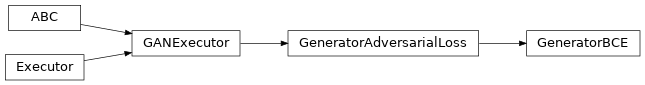# GeneratorBCE¶

Inheritance Diagramclass ashpy.losses.gan.GeneratorBCE(from_logits=True)[source]

The Binary CrossEntropy computed among the generator and the 1 label.

$L_{G} = E [\log (D( G(z))]$

Methods

 __init__([from_logits]) Initialize the BCE Loss for the Generator.

Attributes

 fn Return the Keras loss function to execute. global_batch_size Global batch size comprises the batch size for each cpu. weight Return the loss weight.
__init__(from_logits=True)[source]

Initialize the BCE Loss for the Generator.

Return type: None